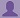To start with, Sr2Jr’s first step is to reduce the expenses related to education. To achieve this goal Sr2Jr organized the textbook’s question and answers. Sr2Jr is community based and need your support to fill the question and answers. The question and answers posted will be available free of cost to all.

# Computer Networking : A Top-down Approach

 Authors: James F. Kurose, Keith W. Ross ISBN: 9780132856201 Edition: 6
 Chapter: Transport Layer Exercise: Problems Question: 47

###Question

Consider the scenario described in the previous problem. Suppose that the 10Mbps link can buffer a finite number of segments. Argue that in order for the link to always be busy sending data, we would like to choose a buffer size that is at least the product of the link speed C and the two-way propagation delay between the sender and the receiver.

###Answer

Let W denote max window size. Let S denote the buffer size. For simplicity, suppose TCP sender sends data packets in a round by round fashion, with each round corresponding to a RTT. If the window size reaches W, then a loss occurs. Then the sender will cut its congestion window size by half, and waits for the ACKs for W/2 outstanding packets before it starts sending data segments again. In order to make sure the link always busying sending data, we need to let the link busy sending data in the period W/(2*C) (this is the time interval where the sender is waiting for the ACKs for the W/2 outstanding packets). Thus, S/C must be no less than W/(2*C), that is, S>=W/2. Let Tp denote the one-way propagation delay between the sender and the receiver. When the window size reaches the minimum W/2 and the buffer is empty, we need to make sure the link is also busy sending data. Thus, we must have W/2/(2Tp)>=C, thus, W/2>=C*2Tp. Thus, S>=C*2Tp.

### Discussions

Post the discussion improve the above solution.